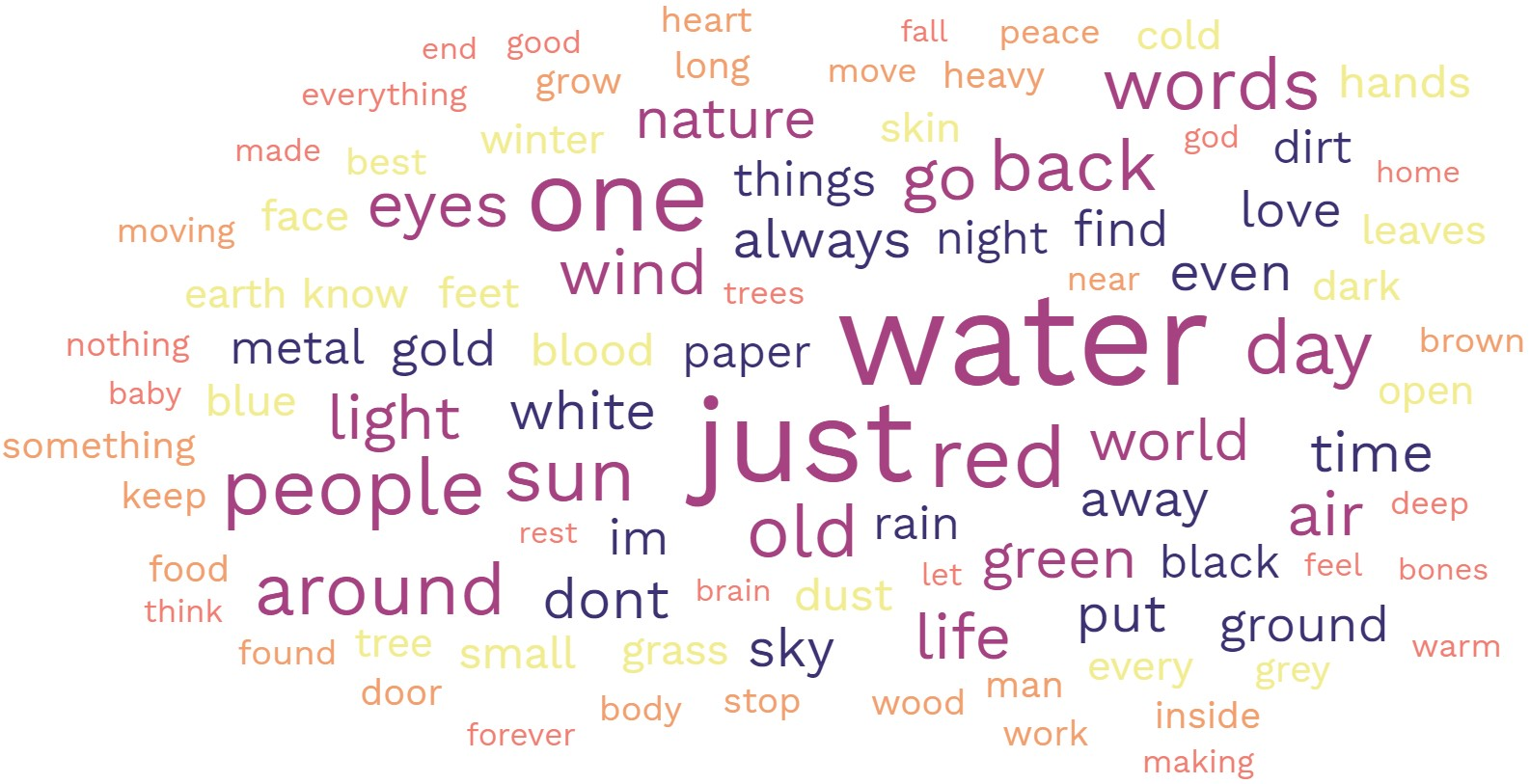# Snowball Poem

6/12/19

I recently learned of an interesting type of poem called "snowball". I have written of these types of poems so far (and will write more!) that you can find here. The basic idea is that the next line in the poem has a word that is one letter longer than the word used in the previous line. In symbols, this is length(linen+1) = length(linen) + 1. Here is an example, my Snowball 1

```oak
tree
nests
abound
holding
birdlike
chickaree
humorously
aggregating
```

For a variation, consider the next line in the poem having a word that is one more syllable longer than the word used in the previous line. That is, syllables(linen+1) = syllables(linen) + 1. An example of this is my Snowball 2

```pines
warble
convulsing
colloquial
echolocations
```

See my spreadsheet here to help create snowball poems. You are free to edit the spreadsheet as needed, but please credit Statisticool.com if you use it. If words are in column A, and syllabes(word) in column B, and length(word) in column C, then the following equations will randomly sample words that have a specified syllable or length.

For example, the following will randomly sample words having 4 letters in them

=INDEX(\$A\$1:\$A\$163919,LARGE(IF(\$C\$1:\$C\$163919=4,ROW(\$C\$1:\$C\$163919)-ROW(\$C\$1)+1),INT(RAND()*COUNTIF(\$C\$1:\$C\$163919,4)+1)))

The following will randomly sample words having 3 syllables

=INDEX(\$A\$1:\$A\$163919,LARGE(IF(\$B\$1:\$B\$163919=3,ROW(\$B\$1:\$B\$163919)-ROW(\$B\$1)+1),INT(RAND()*COUNTIF(\$B\$1:\$B\$163919,3)+1)))

And so on. It is important to not just press Enter when you're done typing these formulas, but press Control-Shift-Enter, to make these formulas array formulas.

These snowball poems can be fun to play with and very challenging to write. To me, they have a similar feeling to Nonet poems. Interestingly, but perhaps not too suprisingly, the correlation between word length and syllables is about .83. You can verify this by using the formula =correl(B:B,C:C) in the spreadsheet. This means that as words get longer they tend to have more syllables, so in some sense snowball poems using word length or syllable count should not feel that different most of the time.